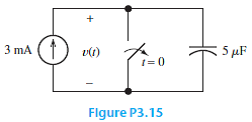Problem

# Find the voltage, power, and stored energy at t = 10 ms for the capacitance in the circu...

Find the voltage, power, and stored energy at t = 10 ms for the capacitance in the circuit of Figure P3.15.#### Step-by-Step Solution

Solution 1

Refer Figure P3.15 in the text book.

For $$t \leq 0$$, the switch in the circuit Figure P3.15 is open. Hence the voltage across the capacitor at $$t=0$$ is,

$$v_{C}(0)=0$$

Calculate the voltage across the capacitor at $$t=10 \mathrm{~ms}$$.

$$v_{C}(t)=\frac{1}{C} \int_{0}^{t} i_{C}(t) d t+v_{C}(0)$$

Substitute 0 for $$v_{C}(0)$$ and $$3 \times 10^{-3}$$ for $$i_{C}(t)$$ and $$5 \times 10^{-6}$$ for $$C$$

\begin{aligned} v_{C}(t) &=\frac{1}{5 \times 10^{-6}} \int_{0}^{10 \times 10^{-3}} 3 \times 10^{-3} d t+0 \\ &=\frac{3 \times 10^{-3}}{5 \times 10^{-6}}[t]_{0}^{10 \times 10^{-3}} \\ &=\frac{3}{5}\left(10^{3} \times 10 \times 10^{-3}\right) \\ &=6 \mathrm{~V} \end{aligned}

Thus, the voltage across the capacitor for this circuit is $$6 \mathrm{~V}$$.

Calculate the power consumed by the capacitor.

\begin{aligned} p &=v_{C}(t) i_{c}(t) \\ &=6\left(3 \times 10^{-3}\right) \\ &=18 \mathrm{~mW} \end{aligned}

Thus, the power consumed by the capacitor is $$18 \mathrm{~mW}$$.

Calculate the energy stored for the capacitor.

\begin{aligned} E &=\frac{1}{2} C v_{C}(t)^{2} \\ &=\frac{1}{2}\left(5 \times 10^{-6}\right)\left(6^{2}\right) \\ &=90 \times 10^{-6} \\ &=0.9 \mathrm{~mJ} \end{aligned}

Thus, the stored energy for the capacitance is $$0.9 \mathrm{~mJ}$$.